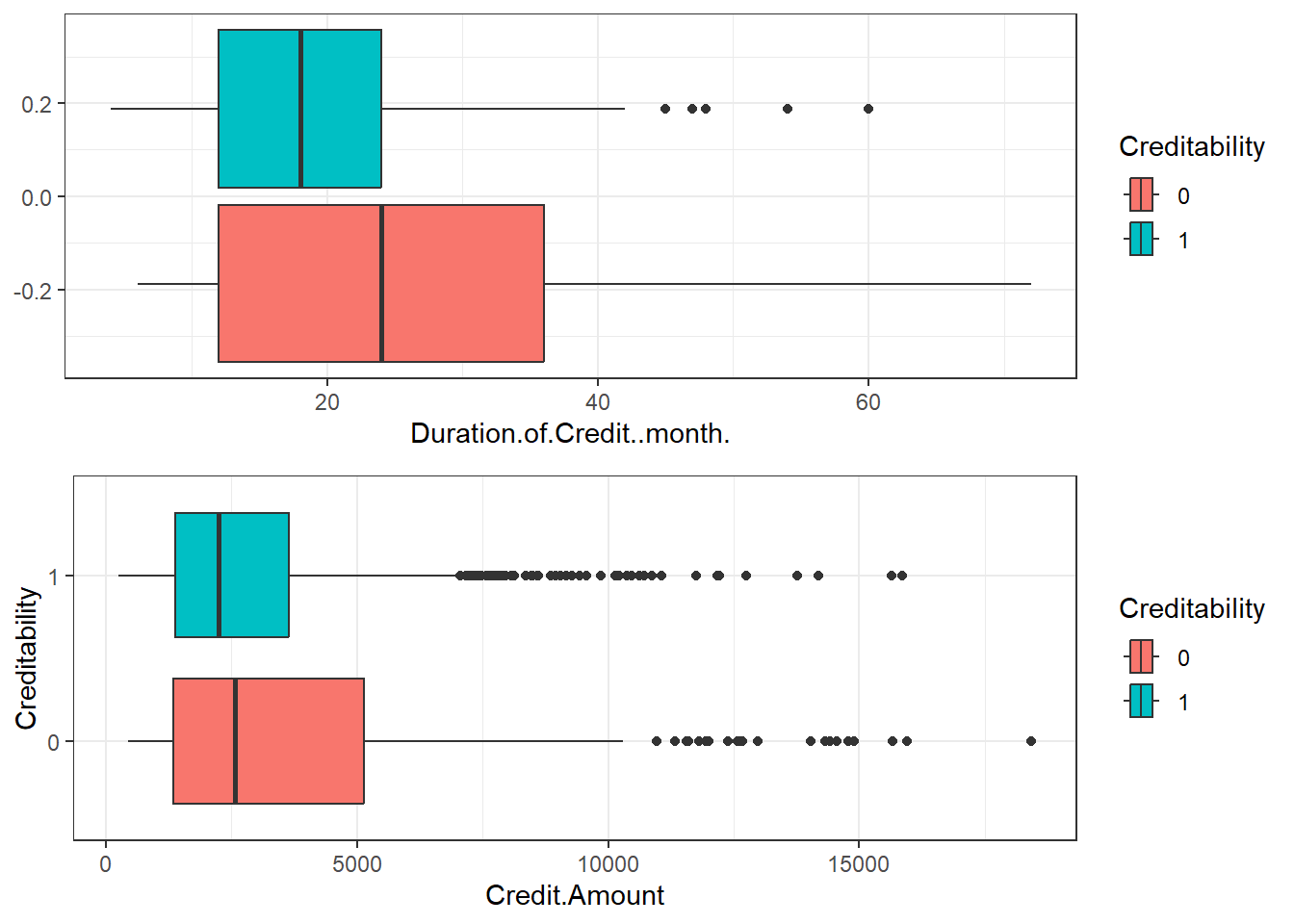## 15.2 Visualisation some features

• Create some visualisation to visualise data characteristics

• Box plots 15.1 for Duration of Credit and Credit Amount

library(ggplot2)
library(gridExtra)
# copy of data for plots
data_cr_p = data_cr
# set theme for the session
theme_set(theme_bw())
p1 = ggplot(data_cr, aes(Duration.of.Credit..month., fill = Creditability)) +
geom_boxplot()
p2 = ggplot(data_cr_p, aes(Credit.Amount, Creditability, fill = Creditability)) +
geom_boxplot()

grid.arrange(p1, p2, ncol = 1)Figure 15.1: Box Plots

• See the relationship between Purpose, Sex/status and Creditability 15.2
p3 = ggplot(data_cr_p, aes(Purpose)) + geom_bar(aes(fill = Creditability),
stat = "count", position = "dodge")

p4 = ggplot(data_cr_p, aes(Sex...Marital.Status)) + geom_bar(aes(fill = Creditability),
stat = "count", position = "dodge")

grid.arrange(p3, p4, ncol = 2)Figure 15.2: Bar plots